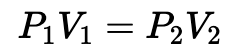# Help Zone

### Student Question

At 25 degrees Celcius, the volume of a gas is 4,6L at a pressure of 98 kPa. What will be its volume if we triple its pressure without changing its temperature?

Chemistry{t c="richEditor.description.title"} {t c="richEditor.description.paragraphMenu"} {t c="richEditor.description.inlineMenu"} {t c="richEditor.description.embed"}

## Explanations (1)

•Explanation from AlloprofExplanation from Alloprof

This Explanation was submitted by a member of the Alloprof team.

Hey!

Thanks for reaching out! Let me help you.

The first step to solve this problem is to calculate the pressure when we triple the initial pressure. Then, as we know that the temperature does not change and that we know the initial volume and the initial volume, we may apply the Boyle-Mariotte rule.

The Boyle-Mariotte rule stipulates that, at a constant temperature, the volume of a gas is inversely proportional to its pressure.

In fact, it leads to the following formula where p1 = initial pressure (kPa or mm Hg), v1 = initial volume (mL or L), p2 = final pressure (kPa or mm Hg), v2 = final volume (mL or L)Hoe that helps!

If there's anything else, you know where to reach us.

Emilie